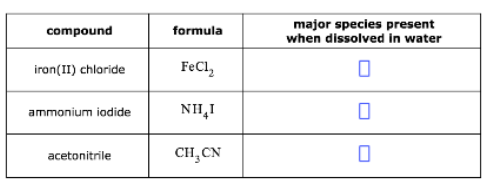# Problem: The names and chemical formulae of some chemical compounds are written in the first two columns of the table below. Each compound is soluble in water. Imagine that a few tenths of a mole of each compound is dissolved in a liter of water. Then, write down in the third column of the table the chemical formula of the major chemical species that will be present in this solution. For example, you know water itself will be present, so you can begin each list with the chemical formula for water (H2O). Note: "major" chemical species are those present in concentrations greater than 10-6 mol/L.

###### FREE Expert Solution

We are being asked to determine the major chemical species that will be present in the following solution

Ionic compounds dissociate in water to its component ions

For this problem, we need the solubility rules:

• Soluble Ionic Compounds:

• Group 1A ions (Li+, Na+, K+, etc.) and Ammonium ion (NH4+) are soluble

• Nitrates (NO3), Acetates (CH3COO or C2H3O2), and most Perchlorates (ClO4) are soluble

• Halides (Cl, Br, and I) are soluble except when paired with Ag+, Pb2+, Cu+, Hg22+

• Sulfates (SO42–) are soluble except those of Ca2+, Sr2+, Ba2+, Ag+, and Pb2+

• Insoluble Ionic Compounds:

• Hydroxides (OH) and Sulfides (S2–) are insoluble except when with Group 1A ions (Li+, Na+, K+, etc.), ammonium ion (NH4+), Ca2+, Sr2+, and Ba2+

• Carbonates (CO32–) and Phosphates (PO43–) are insoluble except when with Group 1A ions (Li+, Na+, K+, etc.) and ammonium ion (NH4+)

90% (167 ratings)###### Problem Details

The names and chemical formulae of some chemical compounds are written in the first two columns of the table below. Each compound is soluble in water. Imagine that a few tenths of a mole of each compound is dissolved in a liter of water. Then, write down in the third column of the table the chemical formula of the major chemical species that will be present in this solution. For example, you know water itself will be present, so you can begin each list with the chemical formula for water (H2O). Note: "major" chemical species are those present in concentrations greater than 10-6 mol/L.Frequently Asked Questions

What scientific concept do you need to know in order to solve this problem?

Our tutors have indicated that to solve this problem you will need to apply the Solubility Rules concept. You can view video lessons to learn Solubility Rules. Or if you need more Solubility Rules practice, you can also practice Solubility Rules practice problems.